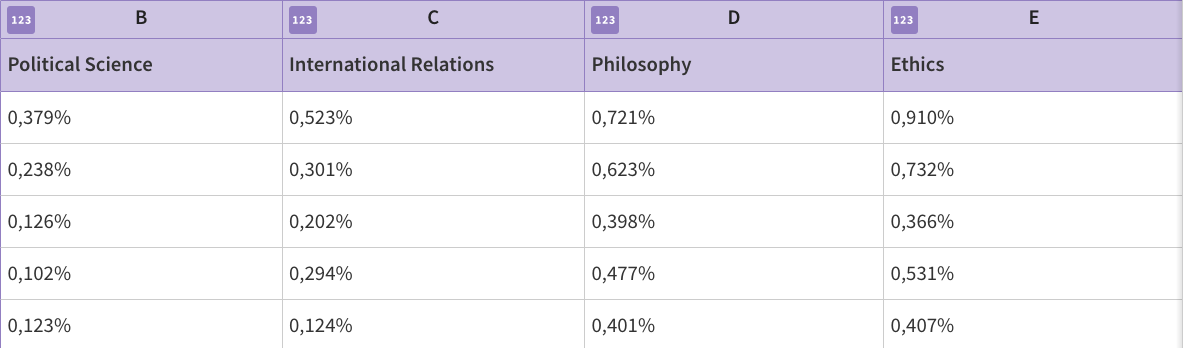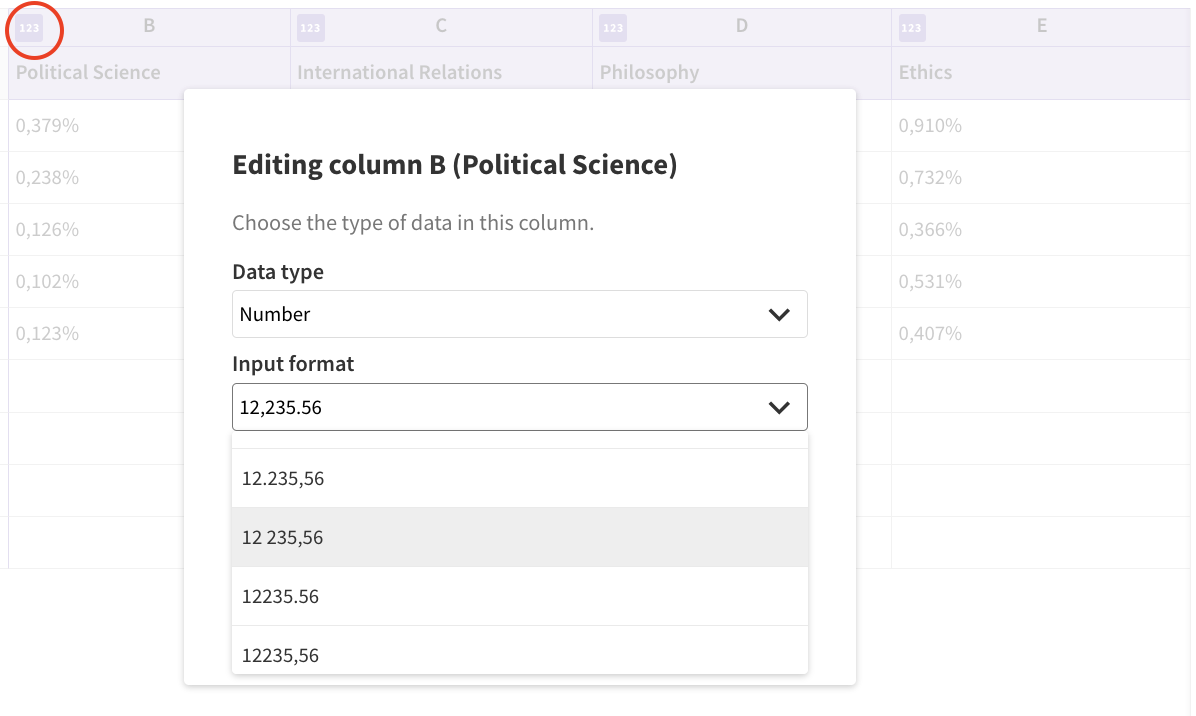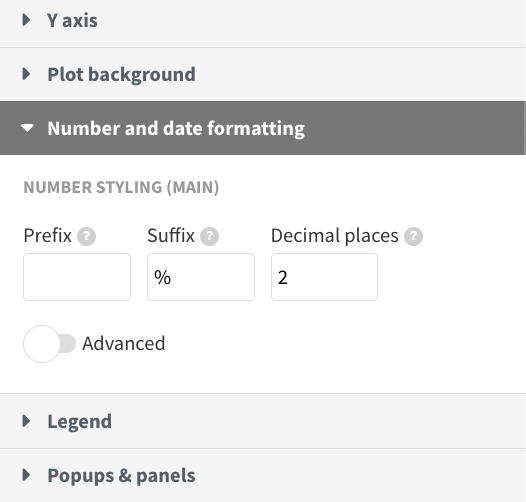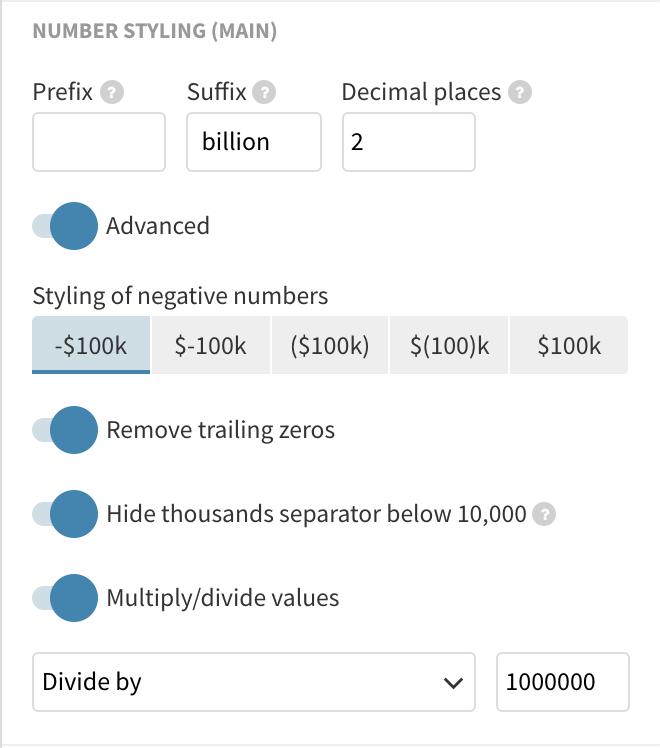# How to change number formatting

#### How to add a prefix or suffix

Are you trying to represent percentages but instead of 2,152% – as you added your numbers in the data – your visualization is showing 2152 as a value?

You will need to change the number format.

1
Switch to the Data tab and check what type of decimal separator your data uses. In this example, it's a comma.2
Click on the data typing button in the top right corner of the column. (This is circled in red below) You can change the input format. I want to change the decimal separator to a comma. You can also change the output format if you wish.3
If you would like to display your number as a percentage, you will have to add a suffix. You can do this under Number styling > Suffix.#### How to multiply or divide values

When working with data with large numbers, you might want to round them to thousands, millions, or billions. You can achieve this with our number formatting settings.

1
Go to the number formatting settings and choose Advanced > Multiply/divide values.You can choose between:
• Multiply by: Multiplies all your values by a certain value
• Divide by: Divides all your values by a certain value
Example: Dividing 1.94E+09 by 1000000000 will return 1.94
• x 10 to the power of: Instead of having lots of zeros, you show how many powers of 10 will make that many zeroes. When supplying a negative number, it will round to that number of zeroes. For example, -3 will give you thousands, and -6 will give you millions.
Example: Supplying -9 powers of 10 for 1.94E+09 will return 1.94

TIP: Use the x 10 to the power of option to easily display millions or billions without having to calculate what to divide your values by.

2
Add a prefix or a suffix (for example, "billions") under  Number styling >  Suffix.

In our example, the data is supplied in the format 1.94E+09. Through our number formatting options, we can easily display it as billions.